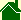#Induction of forces

### Nordberg Interpretation

The same. If a Magnetic field is accelerated, it will induce an Electrical field.

### Nordberg Interpretation

The same. If an Electric field is accelerated, it will induce a Magnetic field.

### Nordberg Interpretation

If an Electrical field and a Magnetic field are both accelerated, the vector cross product of these two fields is an induced gravitational field.

If the Electric and Magnetic fields are in the form of a spherical standing wave, the cross product always points to the center of the sphere.

The cross product -- E cross B equals G -- predicts "force equals mass times acceleration." If you apply a force to a spherical standing wave of electric and magnetic fields -- accelerating the standing wave in any direction -- the acceleration induces greater Electric and Magnetic fields, and thus, a greater cross product.

The cross -- product E cross B equals G -- predicts E = mc2. If an electric field is sweeping over a sphere, it induces a magnetic field at right angles. Integrating the cross product of the two fields -- over the surface of a sphere -- is equivalent energy divided the speed of light squared -- which is equal to mass. (This is a variation of Gauss's law of gravity.)
None